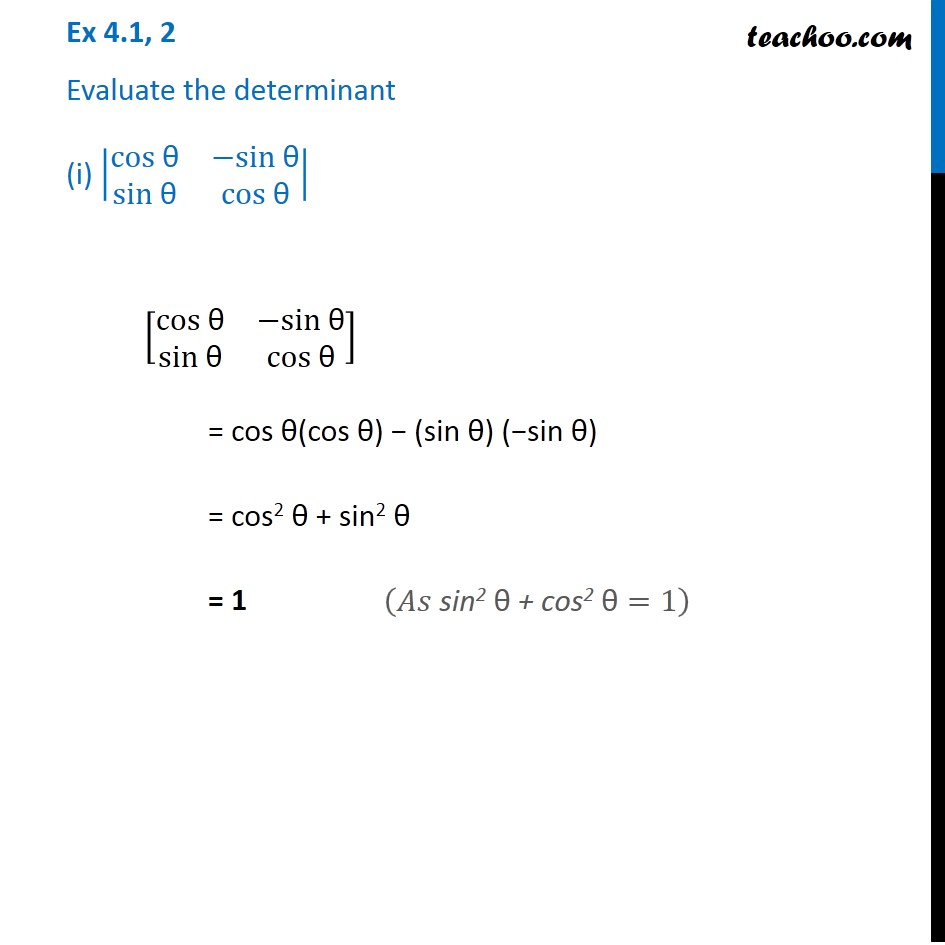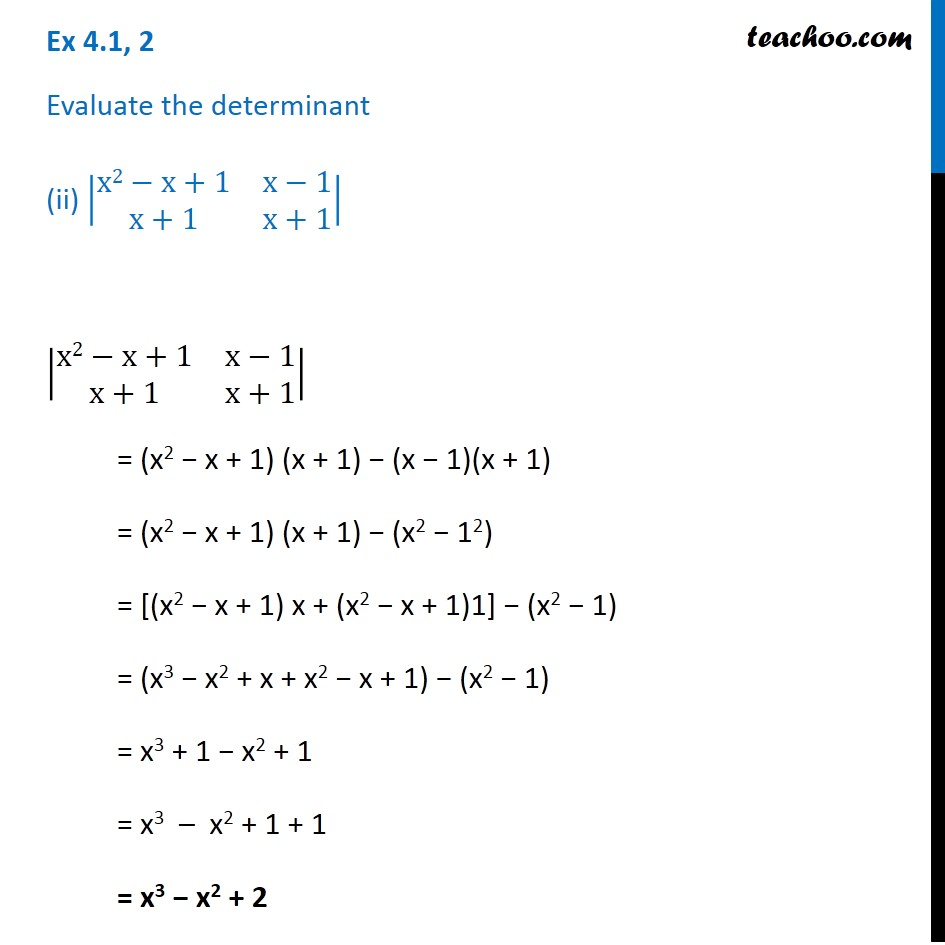Subscribe to our Youtube Channel - https://you.tube/teachoo

1. Chapter 4 Class 12 Determinants
2. Serial order wise
3. Ex 4.1

Transcript

Ex 4.1, 2 Evaluate the determinant (i) |■8(cos "θ" &−sin" θ" @sin "θ" &cos "θ" )| [■8(cos "θ" &−sin "θ" @sin "θ" &cos" θ" )] = cos θ(cos θ) − (sin θ) (−sin θ) = cos2 θ + sin2 θ = 1 (𝐴𝑠 "sin2 θ + cos2 θ" =1) Ex 4.1, 2 Evaluate the determinant (ii) |■8(x2−x+1&x−1@x+1&x+1)| |■8(x2−x+1&x−1@x+1&x+1)| = (x2 − x + 1) (x + 1) − (x − 1)(x + 1) = (x2 − x + 1) (x + 1) − (x2 − 12) = [(x2 − x + 1) x + (x2 − x + 1)1] − (x2 − 1) = (x3 − x2 + x + x2 − x + 1) − (x2 − 1) = x3 + 1 − x2 + 1 = x3 – x2 + 1 + 1 = x3 − x2 + 2

Ex 4.1